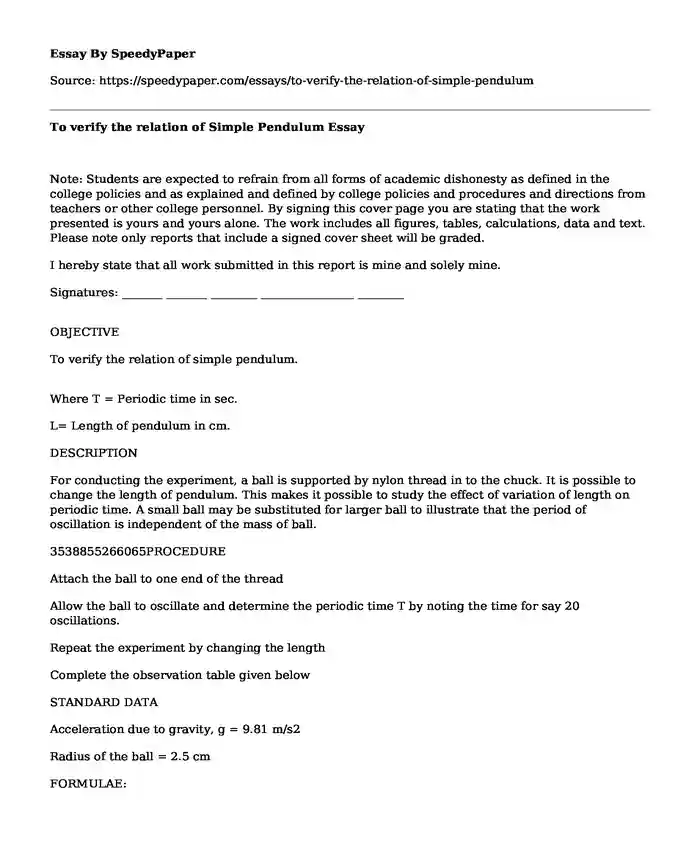# To verify the relation of Simple Pendulum

Published: 2022-12-30Type of paper: Report Categories: Leadership analysis Finance Society World Disorder Pages: 3 Wordcount: 699 words
143 views

Note: Students are expected to refrain from all forms of academic dishonesty as defined in the college policies and as explained and defined by college policies and procedures and directions from teachers or other college personnel. By signing this cover page you are stating that the work presented is yours and yours alone. The work includes all figures, tables, calculations, data and text. Please note only reports that include a signed cover sheet will be graded.

Is your time best spent reading someone else’s essay? Get a 100% original essay FROM A CERTIFIED WRITER!

I hereby state that all work submitted in this report is mine and solely mine.

Signatures: _______ _______ ________ ________________ ________

OBJECTIVE

To verify the relation of simple pendulum.

Where T = Periodic time in sec.

L= Length of pendulum in cm.

DESCRIPTION

For conducting the experiment, a ball is supported by nylon thread in to the chuck. It is possible to change the length of pendulum. This makes it possible to study the effect of variation of length on periodic time. A small ball may be substituted for larger ball to illustrate that the period of oscillation is independent of the mass of ball.

3538855266065PROCEDURE

Attach the ball to one end of the thread

Allow the ball to oscillate and determine the periodic time T by noting the time for say 20 oscillations.

Repeat the experiment by changing the length

Complete the observation table given below

STANDARD DATA

Acceleration due to gravity, g = 9.81 m/s2

Radius of the ball = 2.5 cm

FORMULAE:

Time Period, T Actual =t / nSec.

2181225133985

448881563500Time Period, T Theo. =Sec.

Where

T =Time taken by 'n ' oscillations.

N =Nos. of oscillation. INCLUDEPICTURE "https://i.stack.imgur.com/PFvkb.jpg" \* MERGEFORMATINET

L =Length of pendulum from the center of the ball in cm

OBSERVATION & CALCULATION TABLE:

# Pendulum Length Oscillations Number Oscillations Time Actual Period Theoretical period Error

1 0.66 20 32.72 1.636 1.630 0.34%

2 0.575 20 30.04 1.502 1.522 1.30%

3 0.475 20 28.76 1.438 1.383 3.97%

4 0.38 20 24.49 1.2245 1.237 1.02%

5 0.27 20 20.78 1.039 1.043 0.36%

Calculation sample:

tActual=Ocillations TimeOscillation number= 32.7220=1.636 sectTheoretical=2pLg=2p0.669.81=1.630

Graph: T Theoretical Vs T Actual

Results

Results of counted number of oscillations (20 oscillations) made by the swinging mass were recorded. Also, the time taken during the oscillations was as well recorded.

Final calculations were carried out and the calculated periodic time (T) was found to be approximately 1.363s while the theoretical/ideal periodic time is 1.630s.

DISCUSSION

Following the results obtained there were discrepancies between theoretical and calculated periodic time (T). This might have been caused by various physical factors; human errors (accuracy), this might have been the parallax measurement of the parameters. There might be some errors encountered due to erroneous instruments. For instance, a stopwatch might be the cause of errors instead this errors would be minimized by using the photo sensors which are more precised.

Measurement of amplitude (the length of the string) in a precised and more accurately would also minimize such errors. If a photo timer would be used to measure the periods the sources of these errors would be minimized. Precise devices for this measurements which were to be taken from the laboratory. Besides, there might be systematic errors encountered with during the experiment. The errors might include air resistance of the mass and string producing the oscillations.

Concerning the variable periodic time of which we theoretically know that this measured periodic time should be the same all through since the number of oscillations were 20.

CONCLUSION

In conclusion, we agreed that time was the mostly affected parameter by the variation of string's length. In accordance with the data obtained an analysis of the trend claimed that as the length of the string decreased the periodic time also decreased. The amplitude did not affect time. It was also deduced that the surroundings were not perfect for the experiment thus some friction was experienced by the ball producing the air resistance. The theoretical value is nearly the same to the calculated value thus such minor discrepancies are due to nature, friction and the resistance the air particles to the swinging ball.

References

Nelson, R. A., & Olsson, M. G. (1986). The pendulum-Rich physics from a simple system. American Journal of Physics, 54(2), 112-121.

To verify the relation of Simple Pendulum. (2022, Dec 30). Retrieved from https://speedypaper.com/essays/to-verify-the-relation-of-simple-pendulum

Request Removal

If you are the original author of this essay and no longer wish to have it published on the SpeedyPaper website, please click below to request its removal:

Liked this essay sample but need an original one?

Hire a professional with VAST experience!